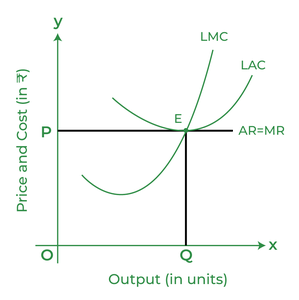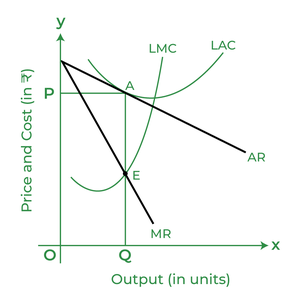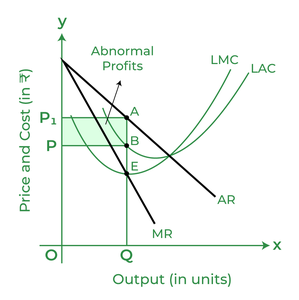Open in App
Not now

# Long-Run Equilibrium under Perfect, Monopolistic, and Monopoly Market

• Last Updated : 20 Jul, 2022

In layman’s language, a Market is a place where the exchange of goods takes place. In other words, a place where the purchase and sale of goods take place is a market. The market is the nervous system of modern economic life where producers and consumers carry out the sale and purchase transactions. The market has a different and wider meaning in Economics, as it does not refer to a specific place. In Economics, a Market is a region where the buyers and sellers do not have to assemble at a specific place for the sale and purchase of goods. Instead, they have to be in contact with each other through any communication means, such as the internet, letter, mail, telephone, etc.

Market refers to the whole region where buyers and sellers of a commodity are in contact with each other to affect the purchase and sale of the commodity.

## Equilibrium

Producer’s equilibrium states that a firm is at equilibrium when it earns maximum profits. As there is freedom of entry in perfect competition and monopolistic competition, the firms can only earn normal profits in the long run. However, as there is a restriction on the entry and exit under a monopoly market, the firms can earn abnormal profits. Therefore, the long-run equilibrium under perfect competition, monopolistic competition, and monopoly are different.

### Long-run Equilibrium under Perfect Competition

Due to freedom of entry and exit into the perfectly competitive market, the firms earn normal profits in the long run. Therefore, AR and MR under perfect competition are equal, and both coincide in a straight horizontal line and are parallel to the X-axis.In the above graph, LMC and LAC are the Long-run Marginal Cost Curve and Long-run Average Cost Curve, respectively. To attain equilibrium, the conditions i) MR = LMC ii) LMC curve cuts the MR curve from below, are fulfilled at point E. Therefore, point E is the equilibrium point of the firm at which it sells equilibrium output OQ at the equilibrium price of OP. Besides this, the LAC of the firm is equal to its AR. Therefore, the firm will only earn normal profits.

### Long-run Equilibrium under Monopolistic Competition

Due to freedom of entry and exit into the monopolistic competition market, the firms earn normal profits in the long run. Also, as the firms under Monopolistic Competition can sell more output by reducing the price of the product, its demand curve or AR curve slopes downward, and because of this, the MR curve also slopes negatively.In the above graph, LMC and LAC are the Long-run Marginal Cost Curve and Long-run Average Cost Curve, respectively. To attain equilibrium, the conditions i) MR = LMC ii) LMC curve cuts the MR curve from below, are fulfilled at point E. Therefore, point E is the equilibrium point of the firm at which it sells equilibrium output OQ at the equilibrium price of OP. Besides this, the LAC of the firm is equal to its AR. Therefore, the firm will only earn normal profits.

### Long-run Equilibrium under Monopoly

Due to restrictions on the entry and exit into the monopoly market, the firms earn abnormal profits in the long run. Also, as the firms can sell more outputs by reducing the price of the product, the demand curve or AR curve of the firm slopes downwards, and because of this the MR curve also slopes negatively.In the above graph, LMC and LAC are the Long-run Marginal Cost Curve and Long-run Average Cost Curve, respectively. To attain equilibrium, the conditions i) MR = LMC ii) LMC curve cuts the MR curve from below are fulfilled at the OQ output level. If the output of the firm is priced at OP1 and LAC at OP, then it will earn abnormal profits of PBAP1

My Personal Notes arrow_drop_up
Related Articles